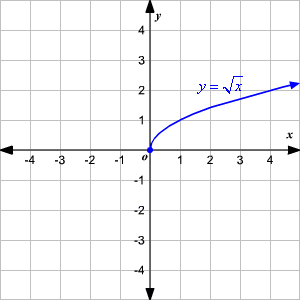The term "square root" usually refers to the definition:

• A number which when squared gives the desired result.
By convention, when we take the square root of a real number the result (if it exists) is positive (or non-negative).

Thus:

• The square root of 9 is 3
• by convention, not -3, even though $(-3)^2=9$
• The square root of 0 is 0.
• There is no square root of -4
We can take the square root of negative real numbers by using imaginary numbers.

When we take the square root of a complex number there is no concept of "positive" so the convention fails and we must then consider both square roots.

By restricting the square root of positive real numbers to the positive result, the square root operation is a function:

 $\sqrt{}:\mathbb{R}^+\mapsto\mathbb{R}^+$The domain of the function is the non-negative real numbers, and (using our convention) the image is also the non-negative real numbers.

When taking the square root of a complex number we have more of a problem. The square root is now defined for every complex number, but every complex number (except 0) has two square roots. That means the square root operation is not a function. Trying to choose one of the square roots over the other doesn't solve the problem completely satisfactorily.

It's nice for functions to be continuous, with the result varying slowly as we vary the input. However, consider the square root of 9. Choose one of the roots, say 3. Now move the input point around in a circle of radius 9 anti-clockwise and the square root also moves in a circle anti-clockwise, but only half as fast. As the input point gets to -9 the square root is $3i.$ By the time the input point gets back to its starting point of 9, the output point, the square root, has only got as far as -3. Arbitrarily choosing just one of the square roots means the result cannot be continuous.

We can solve this by using a Riemann Surface, and more generally, operations that have more than one possible answer can be made one-to-one by using a Riemann surface as the CoDomain. (See CoDomain of a function)

ArgandDiagram
BasinOfAttraction
ComplexNumber
ComplexPlane
CubeRoot
FundamentalTheoremOfAlgebra
GerolamoCardano
NewtonsMethod
RootsOfPolynomials
Logarithm
TypesOfNumber
A4Paper
AGentleIntroductionToNPC
CancellingFractions
CommonFactor
ContinuedFraction
DedekindCut
DomainOfAFunction
EIsIrrational
Euclid
MathematicsTaxonomy
ModuloArithmetic
PiIsIrrational
Pythagoras
RationalNumber
RationalisingTheDenominator
RealNumber
ScalarProduct
TimeComplexity
UnitVector
ImaginaryNumber
RiemannSurface
Root
AGentleIntroductionToTimeComplexity
AbsoluteValue
AlgebraicNumber
EuclideanAlgorithm
InverseFunction
IrrationalNumber
MagnitudeOfAVector
RootTwoIsIrrational
Surd

## You are here

SquareRoot
CoDomainOfAFunction
ComplexNumber
DomainOfAFunction
Function
ImageOfAFunction
RealNumber
BernhardRiemann
CubeRoot
FundamentalTheoremOfAlgebra
HolomorphicFunction
Logarithm
NewtonsMethod
RiemannHypothesis
AlgebraicNumber
ArgandDiagram
CategoryMetaTopic
CauchySequence
ComplexConjugate
ContinuedFraction
DedekindCut
DividingComplexNumbers
Euler
Integer
IrrationalNumber
MultiplyingComplexNumbers
PolarRepresentationOfAComplexNumber
Polynomial
RangeOfAFunction
RationalNumber
TranscendentalNumber